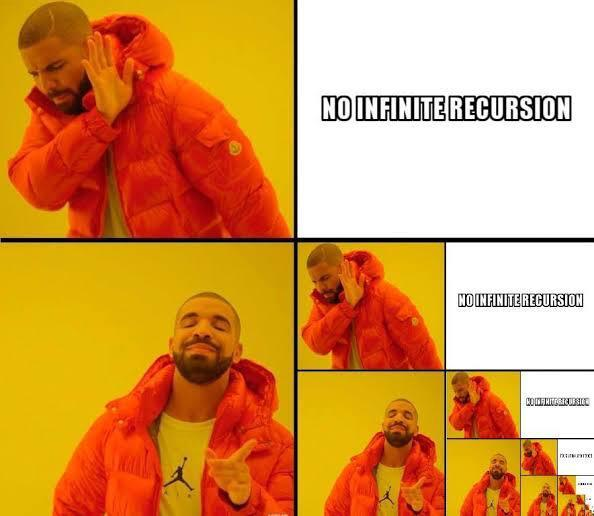Related Articles
Recursion in Python
• Difficulty Level : Easy
• Last Updated : 28 Jul, 2020

The term Recursion can be defined as the process of defining something in terms of itself. In simple words, it is a process in which a function calls itself directly or indirectly.• A complicated function can be split down into smaller sub-problems utilizing recursion.
• Sequence creation is simpler through recursion than utilizing any nested iteration.
• Recursive functions render the code look simple and effective.

• A lot of memory and time is taken through recursive calls which makes it expensive for use.
• Recursive functions are challenging to debug.
• The reasoning behind recursion can sometimes be tough to think through.

Syntax:

```def func(): <--
|
| (recursive call)
|
func() ----
```

Example 1:
A Fibonacci sequence is the integer sequence of 0, 1, 1, 2, 3, 5, 8....

 `# Program to print the fibonacci series upto n_terms`` ` `# Recursive function``def` `recursive_fibonacci(n):``   ``if` `n <``=` `1``:``       ``return` `n``   ``else``:``       ``return``(recursive_fibonacci(n``-``1``) ``+` `recursive_fibonacci(n``-``2``))``  ` `n_terms ``=` `10``  ` `# check if the number of terms is valid``if` `n_terms <``=` `0``:``   ``print``(``"Invalid input ! Please input a positive value"``)``else``:``   ``print``(``"Fibonacci series:"``)``   ``for` `i ``in` `range``(n_terms):``       ``print``(recursive_fibonacci(i))`

Output:

```Fibonacci series:
0
1
1
2
3
5
8
13
21
34
```

Example 2:
The factorial of 6 is denoted as 6! = 1*2*3*4*5*6 = 720.

 `# Program to print factorial of a number ``# recursively.`` ` `# Recursive function``def` `recursive_factorial(n):  ``   ``if` `n ``=``=` `1``:  ``       ``return` `n  ``   ``else``:  ``       ``return` `n ``*` `recursive_factorial(n``-``1``)  `` ` `# user input``num ``=` `6`` ` `# check if the input is valid or not``if` `num < ``0``:  ``   ``print``(``"Invalid input ! Please enter a positive number."``)  ``elif` `num ``=``=` `0``:  ``   ``print``(``"Factorial of number 0 is 1"``)  ``else``:  ``   ``print``(``"Factorial of number"``, num, ``"="``, recursive_factorial(num)) `

Output:

`Factorial of number 6 = 720`

## What is Tail-Recursion?

A unique type of recursion where the last procedure of a function is a recursive call. The recursion may be automated away by performing the request in the current stack frame and returning the output instead of generating a new stack frame. The tail-recursion may be optimized by the compiler which makes it better than non-tail recursive functions.

Is it possible to optimize a program by making use of a tail-recursive function instead of non-tail recursive function?
Considering the function given below in order to calculate the factorial of n, we can observe that the function looks like a tail-recursive at first but it is a non-tail-recursive function. If we observe closely, we can see that the value returned by Recur_facto(n-1) is used in Recur_facto(n), so the call to Recur_facto(n-1) is not the last thing done by Recur_facto(n).

 `# Program to calculate factorial of a number``# using a Non-Tail-Recursive function. `` ` `# non-tail recursive function``def` `Recur_facto(n): ``   ` `    ``if` `(n ``=``=` `0``): ``        ``return` `1``   ` `    ``return` `n ``*` `Recur_facto(n``-``1``) ``   ` `# print the result``print``(Recur_facto(``6``))`

Output:

`720`

We can write the given function Recur_facto as a tail-recursive function. The idea is to use one more argument and in the second argument, we accommodate the value of the factorial. When n reaches 0, return the final value of the factorial of the desired number.

 `# Program to calculate factorial of a number``# using a Tail-Recursive function.`` ` `# A tail recursive function ``def` `Recur_facto(n, a ``=` `1``): ``   ` `    ``if` `(n ``=``=` `0``): ``        ``return` `a ``   ` `    ``return` `Recur_facto(n ``-` `1``, n ``*` `a) ``   ` `# print the result``print``(Recur_facto(``6``))`

Output:

`720`

Attention geek! Strengthen your foundations with the Python Programming Foundation Course and learn the basics.

To begin with, your interview preparations Enhance your Data Structures concepts with the Python DS Course. And to begin with your Machine Learning Journey, join the Machine Learning - Basic Level Course

My Personal Notes arrow_drop_up